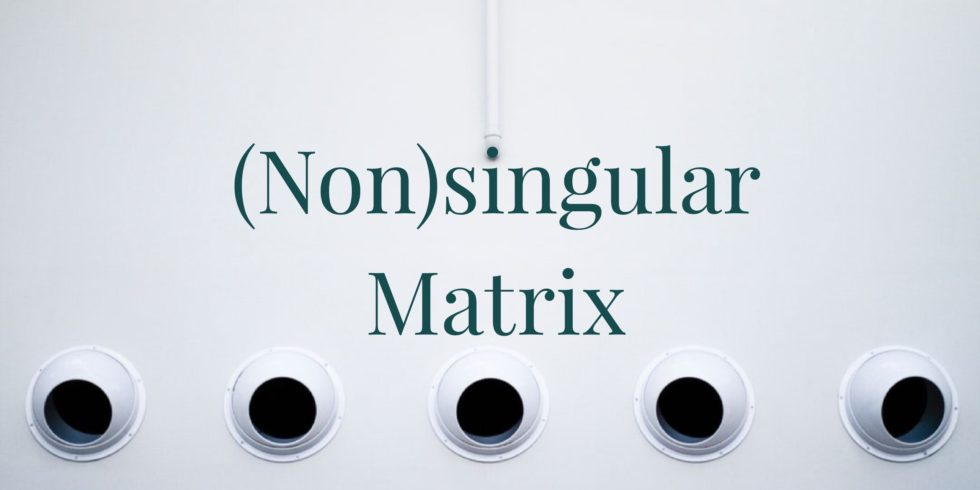# Are Coefficient Matrices of the Systems of Linear Equations Nonsingular?## Problem 669

(a) Suppose that a $3\times 3$ system of linear equations is inconsistent. Is the coefficient matrix of the system nonsingular?

(b) Suppose that a $3\times 3$ homogeneous system of linear equations has a solution $x_1=0, x_2=-3, x_3=5$. Is the coefficient matrix of the system nonsingular?

(c) Let $A$ be a $4\times 4$ matrix and let
$\mathbf{v}=\begin{bmatrix} 1 \\ 2 \\ 3 \\ 4 \end{bmatrix} \text{ and } \mathbf{w}=\begin{bmatrix} 4 \\ 3 \\ 2 \\ 1 \end{bmatrix}.$ Suppose that we have $A\mathbf{v}=A\mathbf{w}$. Is the matrix $A$ nonsingular?Add to solve later

## Hint.

Recall the following fact.

Let $A$ be an $n\times n$ matrix.

Then $A\mathbf{x}=\mathbf{b}$ has a unique solution for every $n\times 1$ column vector $\mathbf{b}$ if and only if $A$ is nonsingular.

## Solution.

### (a) Suppose that a $3\times 3$ system of linear equations is inconsistent. Is the coefficient matrix of the system nonsingular?

Let $A\mathbf{x}=\mathbf{b}$ be the system, where $A$ is the coefficient matrix and $\mathbf{b}$ is the constant term vector. Because this system is inconsistent, then $A$ is singular. (If $A$ would be nonsingular, then the system has a unique solution.)

### (b) Suppose that a $3\times 3$ homogeneous system of linear equations has a solution $x_1=0, x_2=-3, x_3=5$. Is the coefficient matrix of the system nonsingular?

Recall that a homogeneous system of linear equations has always the zero solution. As the system has another solution $x_1=0, x_2=-3, x_3=5$, the system $A\mathbf{x}=\mathbf{0}$ must have infinitely many solutions. Here $A$ is the coefficient matrix.
Thus, the coefficient matrix $A$ is singular. (If $A$ would be nonsingular, the system has only one solution, which must be the zero solution.)

### (c) Suppose that we have $A\mathbf{v}=A\mathbf{w}$. Is the matrix $A$ nonsingular?

Because $A\mathbf{v}=A\mathbf{w}$, we have
$A(\mathbf{v}-\mathbf{w})=A\mathbf{v}-A\mathbf{w}=\mathbf{0}.$ Note that
$\mathbf{v}-\mathbf{w}=\begin{bmatrix} 1 \\ 2 \\ 3 \\ 4 \end{bmatrix}-\begin{bmatrix} 4 \\ 3 \\ 2 \\ 1 \end{bmatrix}=\begin{bmatrix} -3 \\ -1 \\ 1 \\ 3 \end{bmatrix}$ is a nonzero vector.
This implies that the homogeneous system $A\mathbf{x}=\mathbf{0}$ has infinitely many solutions as we have found a nonzero solution.
Hence, $A$ is singular.Add to solve later

### More from my site

#### You may also like...

This site uses Akismet to reduce spam. Learn how your comment data is processed.

###### More in Linear Algebra##### Solving a System of Differential Equation by Finding Eigenvalues and Eigenvectors

Consider the system of differential equations \begin{align*} \frac{\mathrm{d} x_1(t)}{\mathrm{d}t} & = 2 x_1(t) -x_2(t) -x_3(t)\\ \frac{\mathrm{d}x_2(t)}{\mathrm{d}t} & = -x_1(t)+2x_2(t) -x_3(t)\\...

Close